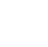Back to Chapter
C++ Setting UpC++ IntroductionNo items found.
C++ BasicsNo items found.
C++ Control StatementsNo items found.
C++ Logical OperatorsNo items found.
C++ Procedural ProgrammingNo items found.
C++ Structural ProgrammingNo items found.
C++ Implementation of OOPSNo items found.
C++ Arrays and VectorsNo items found.
C++ Error HandlingNo items found.
C++ File HandlingNo items found.

# C++ Primitive Data Types

Data types are declarations for variables, data types are used to tell variables the type of data they are used to store. So when we write the following code:

``` int a = 20; ```

we tell the compiler that we want variable "a" to be of "integer" data type. The compiler tells the computer to look for free space in memory and assigns the value 20 to an empty location lets say this location is 1000. The size of an integer in C++ is 4 bytes so 1000 to 1003 will store 20 and say we wish to store another variable of double data type we write the following code:

``` double b = 30; ```

size of a double data type is 8 bytes, therefore, the compiler will tell the computer to look for the next free location after 1001 if 1004 is empty then 1005 1006....1011 will hold the value 30.

A compiler is a special program that processes statements written in a particular programming language and turns them into machine language or "code" that a computer's processor uses. Machine code is binary code(0's and 1's), everything we do on the computer is converted to this code all instruction input basically whatever you see on your screen is stored in computer as 0's and 1's.

The variables a and b are like containers which store the values for later use by programmers.

C++ data types are divided into 3 major categories let us see what these are in the flow chart given below.

##### Primitive data types are built-in data types. These are basic data types that can be directly used to declare variables. Primitive types are the basic building blocks for any programming language and they provide a  base for more complex data types. In C++, each variable has a specific data type, data type tells us the size, range, and the type of value that can be stored in a variable.

Let us look at the primitive data types C++ provides us with.

Integer

Integer data-type as the name suggests is used for the declaration of variables that will contain -ve and +ve integer values. Each variable of int type will take up 4 bytes of the computer memory.

``` int memory = 4; ```

Float

Float data-type is used for the declaration of variables that will contain +ve and -ve integers as well fraction values. Each variable of float will use 4 bytes of the computer's memory.

``` float memory=4.0; ```

Double

double data-type is similar to float but has a larger range and takes up 8 bytes of computer memory.

``` double memory=8.0; ```

Character

Character data type is used to declare single characters. Characters should always be declared inside single quotes or double-quotes. A char variable takes up 1 byte of memory.

``` char memory= "1"; ```

Fun task: copy-paste the following code in your Dev C++ and change the values of char variable a to learn ASCII values.

``` #include using namespace std; main() { char a =1; } ```
ASCII-abbreviated from American Standard Code for Information Interchange is a character encoding standard for electronic communication. ASCII codes represent text in computers. Basically, it converts characters into numbers.Alphabets like 'a', 'b', 'c' ......'z' into 097, 098, 099......122 and 'A', 'B', 'C'........'Z' are represented as 065,066,067.......090. Other special characters are also converted into ASCII codes ranging from 0 to 127.

Booleans

Booleans hold either a true or false value. Keyword bool is used to declare a boolean variable. Have a look at the code given below.

``` bool isRaining = true; ```

Void

Another primitive data type offered by C++, void basically means null. This means void has no value.

``` void example_func() { cout<<"Void does not Return any value"; } ```